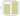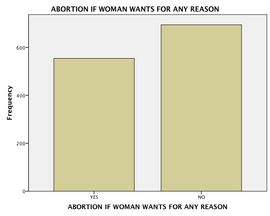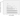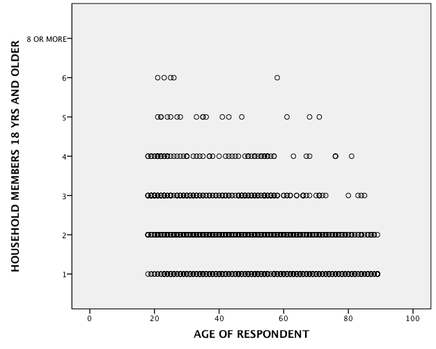# 2012 General Social Survey 4 variable report

Here is a description of the assignment, and my answers are below the assignment.

# Statistics for Social Research SOC310/SWK310

Fall 2014

Final Examination

## DUE DATE: This exam is due on Thursday, December 18, by 5:00 p.m. Remember, exams will not be accepted late, unless you are struck by an incapacitating illness or injury. Even then, I will want to see evidence of just how far you got on the exam before you were stricken.

As with the mid­term, you will be fine provided you plan ahead carefully and leave yourself plenty of time to complete the exam. The computer analysis may take anywhere from fifteen minutes to two or three hours, while it will take several more hours to answer all the questions.

## GENERAL INSTRUCTIONS: For this exam you will be working with four or more variables from the 2012 General Social Survey. A portion of the data from this survey is available on the class web page in a file named gss12.sav. At least two of your variables must be categorical in nature (i.e., either nominal or ordinal), while at least two others must be interval or ratio.

Before anything else, you should check your variables to make sure that values that should be treated as missing have indeed been defined as such in SPSS. You will then describe your variables and test the relationships between certain of them based on information obtained from the FREQUENCIES, CROSSTABS, TTEST, MEANS, SCATTERPLOT and REGRESSION commands.

In addition to your answers to the questions that follow, you will need to turn in all of the relevant output generated by SPSS. Be sure to get rid of any output that is not relevant to the analyses that you have conducted to answer the exam questions. Take care also to document which specific section(s) of your output you are referring to as you answer each question.

## QUESTIONS: As you work through this exam, you will be writing a report on the data and your findings. Such a report typically includes graphs, statistical tables, and text. The text is critical insofar as it highlights, interprets, and summarizes what the graphs and statistics reveal. Answer the following questions in order and in prose form. Report and interpret fully the values of relevant statistics in the text. Try to come as close to a real­world, non­technical explanation of the meaning of each number as possible. And as always, do not forget to label your numbers wherever appropriate.

1. For starters:

1. What population does your sample represent?

2. How large is your sample?

3. How was the sample drawn?

2. For each of your variables:

1. What is the name of each variable in SPSS?

2. What does each variable aim to measure and how is that operationalized?

3. What level of measurement does each variable achieve? Explain.

3. For one interval/ratio level variable of your choosing:

1. Generate a frequency distribution and a bar chart or histogram for your chosen variable. If appropriate, describe the shape of the distribution.

2. Report and interpret the values for all of the measures of central tendency that are appropriate for your variable.

3. Report and interpret the values for all of the measures of dispersion that are appropriate for your variable.

4. For your two categorical variables:

1. State a research hypothesis concerning the relationship between the two categorical variables you have chosen. In doing so, specify theoretically which variable is the independent variable and which is the dependent variable, as well as why you expect there to be a relationship between the two of them.

2. Generate a cross­classification table for the two variables.

3. Describe the pattern and size of any observed relationship between your two variables by making the appropriate percentage comparisons.

4. Test the hypothesis that there is a relationship between the two variables in the population from which the sample is drawn.

5. Report and interpret an appropriate chi­square based measure of association assessing the strength of the observed relationship. Explain why you chose the measure of association you did.

6. Report and interpret an appropriate PRE measure of association assessing the strength of the observed relationship. Explain why you chose the measure of association you did.

5. For one of your categorical variables and one of your interval/ratio level variables:

1. State a research hypothesis concerning the relationship between the two variables that you have chosen. Be sure to state clearly which is the independent variable, which is the dependent variable, and why you expect there to be a relationship between them.

[NOTE: You have a couple of choices here: if the categorical variable has just two categories, or if you wish to focus on just two of its categories, then your research hypothesis can be of the sort expected in a standard difference of means test (t­test); if the categorical variable has more than two categories (and you wish to treat it that way), then your research hypothesis should be of the sort appropriate for an ANOVA test.]

2. Report the overall mean on the interval/ratio level variable as well as the means on that same variable for each of the sub­groups defined by the categorical variable. What do those sub­group means suggest about whether there is a relationship between your two variables?

3. Test the hypothesis that there is a relationship between the two variables in the population from which the samples are drawn.

4. Assess the strength of this relationship using statistics appropriate to the sort of analysis you have conducted.

6. For your two interval/ratio level variables:

1. Briefly explain which variable is the independent variable, which variable is the dependent variable, and why you expect a relationship between the two.

2. Produce a scatterplot summarizing the relationship between the two variables. Does it look like there is a relationship there? If so, how would you describe what that relationship looks like?

3. Report the coefficients of the OLS regression line describing the relationship between the two variables. Explain what these coefficients tell you.

4. Calculate, by hand, predicted values of the dependent variable for two non­zero values of the independent variable.

5. Test the hypothesis that there is a relationship between the two variables in the population from which the sample is drawn.

6. Give and interpret the values of Pearson’s r and R­squared for this relationship.

1.

a. U.S. Americans

b. 1974 people

c. multistage cluster sampling

2.

a. What is the name of each variable in SPSS?

b. What does each variable aim to measure?

·      abany aims to measure if a female respondent answering this question wants abortion legal for any reason. abany is operationalized by requesting respondents to answer “yes” or “no” in response to the question, “Is there any reason you think abortion should be legal?”.

·      advfront aims to measure how much people think science research is necessary and how much it should be funded by the government. advfront is operationalized by having respondents answer either “strongly agree”, “agree”, “disagree”, or “strongly disagree”, according to their corresponding opinion.

·      adults aims to measure the number of children the respondent has. adults is operationalized by having the respondent enter how many adults above 18 they have in the household.

·      age aims to measure the age of the respondent in years. age is operationalized by having the respondent enter a number for their age.

c.

·      abany achieves nominal measurement by having answers without associated magnitude: yes and no.

·      advfront achieves ordinal measurement by having answers that measure magnitude, but the distance between these measurements is not numeric; there is no numeric difference between agree and disagree.

·      adults achieves ratio measurement by having a meaningful zero value (no adults 18 years and above), and by having numeric differences between answers.

·      age achieves ratio level measurement by having a meaningful zero value (zero means that there is no age, and the person is not yet one year old), and by having numeric differences between answers.

3.

a.

Here is a frequency distribution followed by a bar chart for the abany variable.

 ABORTION IF WOMAN WANTS FOR ANY REASON Frequency Percent Valid Percent Cumulative Percent Valid YES 554 28.1 44.4 44.4 NO 694 35.2 55.6 100.0 Total 1248 63.2 100.0 Missing IAP 666 33.7 DK 40 2.0 NA 20 1.0 Total 726 36.8 Total 1974 100.0b.

 Statistics ABORTION IF WOMAN WANTS FOR ANY REASON N Valid 1248 Missing 726 Mode 2

The mode is a measure of central tendency computed by determining which response is most common. A mode of 2 (no) for abany means that the response “no” was given most frequently.

c.

 Statistics ABORTION IF WOMAN WANTS FOR ANY REASON N Valid 1248 Missing 726 Minimum 1 Maximum 2 Percentiles 25 1.00 50 2.00 75 2.00

Percentile is a measure of dispersion. Here the 25th percentile is 1 (yes), calculated by finding the case 25% of the way up through a list of cases ordered from smallest magnitude to greatest magnitude. 50th percentile and 75th percentile can be calculated the same way. A 25th percentile of 1 (yes) means that 25% of the data has a value of 1 (yes) or less. A 50th percentile of 2 (no) means that 2 (no) is a value equal to or greater than 50% of the data.

# 4. Two Categorical Variables: abany and advfront.

a.     abany and advfront are my two categorical variables. abany is the independent variable. This is because a person’s view on whether or not abortion ought to be legalized is more likely to affect their opinion as to how important science research is. The logic here is that if someone wants to have abortions legalized, they will want better science in order to have smoother abortions, therefore, more money ought to be put into science research.

b.     Crosstab between advfront and abany:

 SCI RSCH IS NECESSARY AND SHOULD BE SUPPORTED BY FEDERAL GOVT * ABORTION IF WOMAN WANTS FOR ANY REASON Crosstabulation ABORTION IF WOMAN WANTS FOR ANY REASON Total YES NO SCI RSCH IS NECESSARY AND SHOULD BE SUPPORTED BY FEDERAL GOVT Strongly agree Count 80 68 148 % within ABORTION IF WOMAN WANTS FOR ANY REASON 27.9% 20.7% 24.0% Agree Count 175 215 390 % within ABORTION IF WOMAN WANTS FOR ANY REASON 61.0% 65.3% 63.3% Disagree Count 23 37 60 % within ABORTION IF WOMAN WANTS FOR ANY REASON 8.0% 11.2% 9.7% Strongly disagree Count 9 9 18 % within ABORTION IF WOMAN WANTS FOR ANY REASON 3.1% 2.7% 2.9% Total Count 287 329 616 % within ABORTION IF WOMAN WANTS FOR ANY REASON 100.0% 100.0% 100.0%

c.     61 percent of women who want abortion legalized agree that science research is necessary, while 65 percent who do not want abortion legalized agree that science research is necessary. This begins to lead me to reject my anticipated results, because there are more women agreeing for science who do not want abortion legalized. However, when looking at the strongly agree row, my hypothesis begins to look true again, because there are 7.2% more abany respondents who said yes than those who said no.

## d.     Test the hypothesis that there is a relationship between the two variables in the population from which the sample is drawn

I.               Assumptions

a.     L of M: 2 nominal

b.     Sampling: random

c.     sample size: 616, 4 x 2 table

d.     Population distributions: N/A

II.             Hypotheses:

a.     Ho = no relationship between groups abany and advfront

b.     Ha = relationship between the two variables

III.           Test Statistic

a.     chi-squared

b.     df = 3

c.     X^2 = 5.504

 Chi-Square Tests Value df Asymp. Sig. (2-sided) Pearson Chi-Square 5.504a 3 .138 Likelihood Ratio 5.515 3 .138 Linear-by-Linear Association 3.177 1 .075 N of Valid Cases 616 a. 0 cells (0.0%) have expected count less than 5. The minimum expected count is 8.39.

IV.          p-value and interpretation

a.     p = .138

b.     If Ho were true, the probability of getting a crosstab like we got is less than .138.

V.            Conclusion

a.     Given p = .138, we fail to reject the Ho and do not find support for Ha.

e. Cramer’s V X^2 based measure of association test. I chose this test because it is based off of X^2, and also is suitable for tables bigger than 2×2, because it corrects a problem that comes up in the Phi value test for tables bigger than 2×2. Here we observe a very weak relationship, because the value of Cramer’s V (0.95) is on the low end of the “weak relationship” scale, which extends from 0 – 0.3.

 Symmetric Measures Value Approx. Sig. Nominal by Nominal Phi .095 .138 Cramer’s V .095 .138 N of Valid Cases 616 a. Not assuming the null hypothesis. b. Using the asymptotic standard error assuming the null hypothesis.

f. Gamma PRE measure of association test. The value of gamma between abany and advfront is .156, which means that we do 15.6% better predicting values of advfront when we know whether the woman respondent thinks abortion should be legalized for any reason than if we don’t. I chose this measure of association because I have an ordinal level variable as well as a nominal level variable. Ordinal measures of association are appropriate for a mixed test like this (one ordinal and one nominal level variable), unless there are more than two options for the nominal level variable (Agresti and Finlay 246).

 Symmetric Measures Value Asymp. Std. Errora Approx. Tb Approx. Sig. Ordinal by Ordinal Gamma .156 .074 2.088 .037 N of Valid Cases 616 a. Not assuming the null hypothesis. b. Using the asymptotic standard error assuming the null hypothesis.

5.

a.     research hypothesis between abany (dependent variable) and age (independent variable):

Ho: There is no relationship between abany  and age. μ1 – μ2 </= 0

Ha: There is a relationship between abany and age. μ1 – μ2 > 0

I expect there to be a relationship between these two variables because as someone gets older, their perspectives tend to change, and there may be a common direction in which the beliefs tilt. Another, more realistic reason is that the older respondents matured during a time when the common belief was against abortion, while younger respondents matured when pro-abortion was a more common belief.

b.     The mean for age is 48.19. For Yes the mean age is 46.63, and for No the mean age is 48.88. There may be a slight relationship between the two variables, but age doesn’t seem to have much affect on abany, because the mean age for No was only 2.25 years different from the mean age for Yes.

 Statistics ABORTION IF WOMAN WANTS FOR ANY REASON AGE OF RESPONDENT N Valid 1248 1969 Missing 726 5 Mean 1.56 48.19

Descriptives

ABORTION IF WOMAN WANTS FOR ANY REASON

Statistic

Std. Error

 Kurtosis -0.918 0.186

AGE OF RESPONDENT

YES

Mean

46.63

.691

95% Confidence Interval for Mean

Lower Bound

45.27

Upper Bound

47.99

5% Trimmed Mean

46.18

Median

46.00

Variance

263.871

Std. Deviation

16.244

Minimum

18

Maximum

89

Range

71

Interquartile Range

24

Skewness

.310

.104

Kurtosis

-.531

.207

NO

Mean

48.88

.689

95% Confidence Interval for Mean

Lower Bound

47.52

Upper Bound

50.23

5% Trimmed Mean

48.45

Median

49.00

Variance

327.119

Std. Deviation

18.086

Minimum

18

Maximum

89

Range

71

Interquartile Range

30

Skewness

.236

.093

c.

I.               Assumptions

a.     L of M: 1 nominal (abany), 1 ratio (age).

b.     M of S: random

c.     Sample size: 1248 valid responses = n (variables from same sample, so there’s only one sample size to report)

d.     Population distribution: N/A

II.             Hypotheses

a.     Ho: there is no relationship between abany and age, μ1 – μ2 </= 0

b.     Ha: there is a relationship between abany and age, μ1 – μ2 > 0

III.           Test

a.

 One-Sample Test Test Value = 0 t df Sig. (2-tailed) Mean Difference 95% Confidence Interval of the Difference Lower Upper ABORTION IF WOMAN WANTS FOR ANY REASON 110.598 1247 .000 1.556 1.53 1.58 AGE OF RESPONDENT 120.908 1968 .000 48.193 47.41 48.98

IV.          p-value and interpretation

a.     p < .000

b.     If Ho were true (μ1 – μ2 </= 0), then the probability of getting a sample difference of means as far above 0 as we got  (2.25 years) is .000.

V.            Conclusion

a.     We reject Ho, therefore, by proof of contradiction, we find support for the alternative Ha (μ1 – μ2 > 0).

d. This relationship is very weak, because the gamma value is .067.

 Symmetric Measures Value Asymp. Std. Errora Approx. Tb Approx. Sig. Ordinal by Ordinal Gamma .067 .033 2.021 .043 N of Valid Cases 1243 a. Not assuming the null hypothesis. b. Using the asymptotic standard error assuming the null hypothesis.

6.

a. My two variables are age (ratio) and adults (ratio). Age is the independent variable, because it affects the amount of adults over age 18 living in one’s household.

b. scatter plot of age (x) vs. adults (y)The younger the respondent the higher the likelihood that they have a greater number of members in their household.

c. The unstandardized coefficient of the age of respondent is -.012, which tells us that there is a negative slope, where for every increase in year of age there is a decrease of .012 in number of people over 18 in one’s household. The unstandardized coefficient under column b, in row constant, has a value of 2.473. This is the y intercept of the graph.

 Coefficientsa Model Unstandardized Coefficients Standardized Coefficients t Sig. B Std. Error Beta 1 (Constant) 2.473 .053 47.022 .000 AGE OF RESPONDENT -.012 .001 -.258 -11.771 .000 a. Dependent Variable: HOUSEHOLD MEMBERS 18 YRS AND OLDER

d.         y = -.012x + 2.473.

y = -.012(21) + 2.473

y = 2.221 people. The number of people over 18 most likely to be in a 21 year old’s household is 2.221.

y = -.012(55) + 2.473

y = 1.813 people. The number of people over 18 most likely to be in a 55 year old’s household is 1.813.

e.

I. assumptions

a. L of M: two ratio (from the same sample): adults and age

b. M of S: random

c. sample size: n = 1958 people

d. assumptions: N/A, b/c of C.L.T. (sample size over 30).

II. hypotheses test:

a. Ho: there is no relationship, population slope = 0

b. Ha: there is a relationship, population slope =/ 0

III. test statistic

a. t = 120.908

 Test Value = 0 t df Sig. (2-tailed) Mean Difference 95% Confidence Interval of the Difference Lower Upper AGE OF RESPONDENT 120.908 1968 .000 48.193 47.41 48.98 HOUSEHOLD MEMBERS 18 YRS AND OLDER 101.007 1957 .000 1.891 1.85 1.93

IV. interpret p-value

a.     a. p < .000

b.     If Ho were true (population slope = 0), then the probability of getting a slope like we got (-.012 household members over 18 per year of age) is .000.

V. conclusion

a.     We reject Ho, therefore, by proof of contradiction, we find support for the alternative Ha (population slope =/ 0).

f. R square is .066. The average error from a straight line that our results deviate is 0.66. The Pearson’s r is -.258., meaning there is a negative relationship, but it is not perfect.

 Model Summary and Parameter Estimates Dependent Variable: HOUSEHOLD MEMBERS 18 YRS AND OLDER Equation Model Summary Parameter Estimates R Square F df1 df2 Sig. Constant b1 Linear .066 138.559 1 1951 .000 2.473 -.012 The independent variable is AGE OF RESPONDENT.

 Correlations HOUSEHOLD MEMBERS 18 YRS AND OLDER AGE OF RESPONDENT HOUSEHOLD MEMBERS 18 YRS AND OLDER Pearson Correlation 1 -.258** Sig. (2-tailed) .000 Sum of Squares and Cross-products 1342.611 -7374.530 Covariance .686 -3.778 N 1958 1953 AGE OF RESPONDENT Pearson Correlation -.258** 1 Sig. (2-tailed) .000 Sum of Squares and Cross-products -7374.530 615657.277 Covariance -3.778 312.834 N 1953 1969 **. Correlation is significant at the 0.01 level (2-tailed).

I wrote this report for Gordon College course, Statistics for Social Research. Find the syllabus below.

Categorization:
Mathematics
,
Sociology